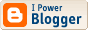<\$BlogRSDURL\$>

The Nacreous Oughts

## A Dissent on Odette

(via phl dot upr dot edu)

The planet Kepler-186f (in Cygnus, which is why i call it "Odette") is being touted as the latest, most likely Earth-analogue yet discovered. While i like for these exoplanets to capture public attention, albeit ill-informedly (there's already talk of "going there"--as if 3 quadrillion miles were a Sunday drive), i have only just made a few preliminary calculations, without having seen the actual scientific paper.

At 492 light years, it's not in the immediate neighborhood. That makes a parallax of something like ".00662; which with an apparent magnitude 14.625 gives a visual luminosity of 0.028038933 solar. This looks to me like a main sequence star of about K8 spectral type. (We'll come back to that question.) When i plug in K8's 3800 K stellar temperature & the corresponding bolometric correction of -0.91, i get a total luminosity of 0.064827829 solar. However, the radius produced by these numbers is 0.59315683, much different from the 0.472 given by my sources. (They say the temperature is 3788, actually.) For the mass given as 0.478, my models suggest a visual luminosity of only 0.008 solar (with that apparent magnitude, the distance would better be at 263 light years.); that should be type M0.5 with a temperature of 3375 K, BC of -1.235, & derived radius 0.87387727 (total luminosity 0.087555618). I would put the mass at 0.52-0.53 instead.

Let's assume the distance is correct. the visual luminosity suggests type K9 (3600 K) BC -1.01 which gives total luminosity 0.071167878 & stellar radius 0.692457296, which i will provisionally use. We know the planet's rotation period is 129.94598 days. When i calculate the planet's semi-major axis from mass 0.52, i get a= 0.403748549 (they say "0.36-0.41"--good agreement). This works out to a blackbody temperature of 227 K.

Finding out the planet's real temperature from there is a matter of further supposition. If it has the same albedo & greenhouse effect as Earth (A 0.36, G 38 C) the average surface temperature is -32 C. That's between Earth & Mars, as we might expect. Assuming a thicker atmosphere (A 0.4375 G 50, A 0.51 G 60) makes it -26 or -23 C. In other words, we can make it a little warmer, but not a lot.

We know a little more; two things cause a reevaluation, to my mind, of our whole picture of this planet. First, the metallicity (log Fe/H) is given as -0.28, or 52% solar. I have long used a rule of thumb, not based on actual physical processes, but which makes it possible to assign a rocky-planet assumed density per the stellar metals. (A star of lower metallicity might produce less dense planets, all other things being equal.) If i adopt, by my rule, a planetary density of 0.616595001 times Earth's, with the observed radius 1.11 the mass will be only 0.84 & the gravity 0.68. So in effect it's a smaller world... Trying a more realistic A 0.2775, G 30 gives the average temperature of -33 C. This is distinctly "Barsoom-like".

It's being assumed too blithely that the rotation of this planet would be synchronous. But its tidal effect, 3.25, comes between Venus & Mercury, neither of which is captured (--Venus if anything is synched to Earth!); some of my calculations provide a likely rotation period of 1/10th its revolution period e.g. 13 days. So a "day" is a week & a "night" is a week. I estimate, with the lesser atmosphere (the actual amount of water being an extreme unknown factor), the diurnal temperature variation being on the order of 43 C either way, from 10 C to -76 C. In sum, a fairly slow rotator, heavy ice caps north & south, considerable day-to-night temperature extremes, with a big (1.7 solar apparent size) red sun in the sky... Odette.

(via kickass.to)

------------------------------------------------------------------------------------------
(Another formula i found gives, irrespective of stellar (& perhaps planetary) metallicity, the radius of a rocky planet is proportional to its mass by the power of 0.26 or 0.27; the derived mass for Odette becomes 1.482620868; then i get a density of 1.0840796 times Earth's, & gravity 1.203328357. Which means the albedo & greenhouse effect, & thus temperature, might be more like the exact earth analogue mentioned previously. Though the diurnal temperature variation would then be less, than the final number i came up with.)

Labels: ,